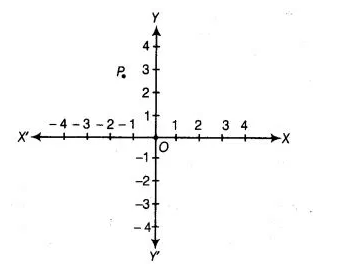# In following figure, coordinates of P are`
Question:

In following figure, coordinates of P are(a) $(-4,2)$

(b) $(-2,4)$

(c) $(4,-2)$

(d) $(2,-4)$

Solution:

(b) Here, given point P lies in II quadrant, so its abscissa will be negative and ordinate wilt be positive. Also, its perpendicular distance from X-axis is 4, so y-coordinate of P is 4 and its perpendicular distance from Y-axis is 2, so x-coordinate is -2. Hence, coordinates of P are (-2, 4).